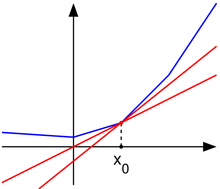次导数 次梯度 小结

.导数(Derivative)的定义

f(x)=limΔx0ΔyΔx=limΔx0f(x0+Δx)f(x0)Δx

f(x)=f(x)f(x0)xx0

2.次导数(subderivative)3.次导数与次微分(subdifferential)计算方式

f(x)f(x0)c(xx0)

a=limxx0f(x)f(x0)xx0

b=limxx+0f(x)f(x0)xx0

4.性质及推广

1.凸函数f:I→R在x0可导，当且仅当次微分只由一个点组成，这个点就是函数在x0的导数。
2.点x0是凸函数f的最小值，当且仅当次微分中包含零，也就是说，在上面的图中，我们可以作一条水平的“次切线”。这个性质是“可导函数在极小值的导数是零”的事实的推广。

5.次梯度

f(x)f(x0)v(xx0)